The OpenFOAM Foundation
IDEA Class Reference

The IDEA fuel is constructed by adding 30% alphaMethylNaphthalene with 70% n-decane. More...

Inheritance diagram for IDEA: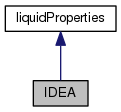[legend]
Collaboration diagram for IDEA:[legend]

## Public Member Functions

TypeName ("IDEA")
Runtime type information. More...

IDEA ()
Construct null. More...

IDEA (const liquidProperties &l, const NSRDSfunc5 &density, const NSRDSfunc1 &vapourPressure, const NSRDSfunc6 &heatOfVapourisation, const NSRDSfunc0 &heatCapacity, const NSRDSfunc0 &enthalpy, const NSRDSfunc7 &idealGasHeatCapacity, const NSRDSfunc4 &secondVirialCoeff, const NSRDSfunc1 &dynamicViscosity, const NSRDSfunc2 &vapourDynamicViscosity, const NSRDSfunc0 &thermalConductivity, const NSRDSfunc2 &vapourThermalConductivity, const NSRDSfunc6 &surfaceTension, const APIdiffCoefFunc &vapourDiffussivity)

IDEA (Istream &is)
Construct from Istream. More...

IDEA (const dictionary &dict)
Construct from dictionary. More...

IDEA (const IDEA &liq)
Construct copy. More...

virtual autoPtr< liquidPropertiesclone () const
Construct and return clone. More...

scalar rho (scalar p, scalar T) const
Liquid density [kg/m^3]. More...

scalar pv (scalar p, scalar T) const
Vapour pressure [Pa]. More...

scalar hl (scalar p, scalar T) const
Heat of vapourisation [J/kg]. More...

scalar Cp (scalar p, scalar T) const
Liquid heat capacity [J/(kg K)]. More...

scalar h (scalar p, scalar T) const
Liquid Enthalpy [J/(kg)]. More...

scalar Cpg (scalar p, scalar T) const
Ideal gas heat capacity [J/(kg K)]. More...

scalar B (scalar p, scalar T) const
Second Virial Coefficient [m^3/kg]. More...

scalar mu (scalar p, scalar T) const
Liquid viscosity [Pa s]. More...

scalar mug (scalar p, scalar T) const
Vapour viscosity [Pa s]. More...

scalar K (scalar p, scalar T) const
Liquid thermal conductivity [W/(m K)]. More...

scalar Kg (scalar p, scalar T) const
Vapour thermal conductivity [W/(m K)]. More...

scalar sigma (scalar p, scalar T) const
Surface tension [N/m]. More...

scalar D (scalar p, scalar T) const
Vapour diffussivity [m2/s]. More...

scalar D (scalar p, scalar T, scalar Wb) const
Vapour diffussivity [m2/s] with specified binary pair. More...

void writeData (Ostream &os) const
Write the function coefficients. More...Public Member Functions inherited from liquidProperties
TypeName ("liquidProperties")

declareRunTimeSelectionTable (autoPtr, liquidProperties,,(),())

declareRunTimeSelectionTable (autoPtr, liquidProperties, Istream,(Istream &is),(is))

declareRunTimeSelectionTable (autoPtr, liquidProperties, dictionary,(const dictionary &dict),(dict))

liquidProperties (scalar W, scalar Tc, scalar Pc, scalar Vc, scalar Zc, scalar Tt, scalar Pt, scalar Tb, scalar dipm, scalar omega, scalar delta)
Construct from components. More...

liquidProperties (Istream &is)
Construct from Istream. More...

liquidProperties (const dictionary &dict)
Construct from dictionary. More...

liquidProperties (const liquidProperties &liq)
Construct copy. More...

virtual ~liquidProperties ()
Destructor. More...

scalar W () const
Molecular weight [kg/kmol]. More...

scalar Tc () const
Critical temperature [K]. More...

scalar Pc () const
Critical pressure [Pa]. More...

scalar Vc () const
Critical volume [m^3/kmol]. More...

scalar Zc () const
Critical compressibilty factor. More...

scalar Tt () const
Triple point temperature [K]. More...

scalar Pt () const
Triple point pressure [Pa]. More...

scalar Tb () const
Normal boiling temperature [K]. More...

scalar dipm () const
Dipole moment []. More...

scalar omega () const
Pitzer's ascentric factor []. More...

scalar delta () const
Solubility parameter [(J/m^3)^(1/2)]. More...

virtual scalar pvInvert (scalar p) const
Invert the vapour pressure relationship to retrieve the. More...

## Friends

Ostreamoperator<< (Ostream &os, const IDEA &l)
Ostream Operator. More...Static Public Member Functions inherited from liquidProperties
static autoPtr< liquidPropertiesNew (Istream &is)
Return a pointer to a new liquidProperties created from input. More...

static autoPtr< liquidPropertiesNew (const dictionary &dict)
Return a pointer to a new liquidProperties created from dictionary. More...

## Detailed Description

The IDEA fuel is constructed by adding 30% alphaMethylNaphthalene with 70% n-decane.

The new properties have been calculated by adding the values in these proportions and making a least square fit, using the same NSRDS-eq. so that Y = 0.3*Y_naphthalene + 0.7*Y_decane

The valid Temperature range for n-decane is normally 243.51 - 617.70 K and for the naphthalene it is 242.67 - 772.04 K The least square fit was done in the interval 244 - 617 K

The critical temperature was taken to be 618.074 K, since this is the 'c'-value in the rho-equation, which corresponds to Tcrit, This value was then used in the fit for the NSRDS6-eq, which uses Tcrit. (important for the latent heat and surface tension)

The molecular weights are 142.20 and 142.285 and for the IDEA fuel it is thus 142.26 ( approximately 0.3*142.2 + 0.7*142.285 )

Critical pressure was set to the lowest one (n-Decane)

Critical volume... also the lowest one (naphthalene) 0.523 m^3/kmol

Second Virial Coefficient is n-Decane

Source files

Definition at line 80 of file IDEA.H.

## Constructor & Destructor Documentation

 IDEA ( )

Construct null.

Definition at line 41 of file IDEA.C.

Referenced by IDEA::clone().

Here is the caller graph for this function: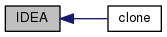IDEA ( const liquidProperties & l, const NSRDSfunc5 & density, const NSRDSfunc1 & vapourPressure, const NSRDSfunc6 & heatOfVapourisation, const NSRDSfunc0 & heatCapacity, const NSRDSfunc0 & enthalpy, const NSRDSfunc7 & idealGasHeatCapacity, const NSRDSfunc4 & secondVirialCoeff, const NSRDSfunc1 & dynamicViscosity, const NSRDSfunc2 & vapourDynamicViscosity, const NSRDSfunc0 & thermalConductivity, const NSRDSfunc2 & vapourThermalConductivity, const NSRDSfunc6 & surfaceTension, const APIdiffCoefFunc & vapourDiffussivity )

Definition at line 117 of file IDEA.C.

 IDEA ( Istream & is )

Construct from Istream.

Definition at line 151 of file IDEA.C.

 IDEA ( const dictionary & dict )

Construct from dictionary.

Definition at line 170 of file IDEA.C.

 IDEA ( const IDEA & liq )

Construct copy.

Definition at line 189 of file IDEA.C.

## Member Function Documentation

 TypeName ( "IDEA" )

Runtime type information.

 virtual autoPtr clone ( ) const
inlinevirtual

Construct and return clone.

Reimplemented from liquidProperties.

Definition at line 141 of file IDEA.H.

Here is the call graph for this function: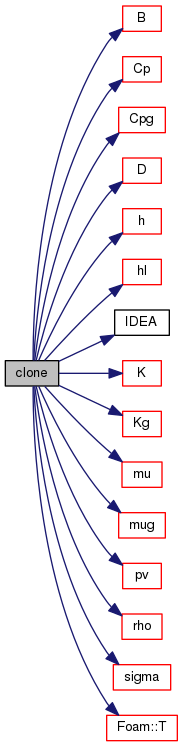Foam::scalar rho ( scalar p, scalar T ) const
inlinevirtual

Liquid density [kg/m^3].

Reimplemented from liquidProperties.

Definition at line 26 of file IDEAI.H.

References NSRDSfunc5::f().

Referenced by IDEA::clone().

Here is the call graph for this function: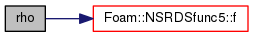Here is the caller graph for this function: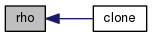Foam::scalar pv ( scalar p, scalar T ) const
inlinevirtual

Vapour pressure [Pa].

Reimplemented from liquidProperties.

Definition at line 32 of file IDEAI.H.

References NSRDSfunc1::f().

Referenced by IDEA::clone().

Here is the call graph for this function: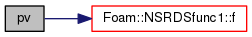Here is the caller graph for this function: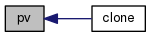Foam::scalar hl ( scalar p, scalar T ) const
inlinevirtual

Heat of vapourisation [J/kg].

Reimplemented from liquidProperties.

Definition at line 38 of file IDEAI.H.

References NSRDSfunc6::f().

Referenced by IDEA::clone().

Here is the call graph for this function: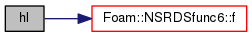Here is the caller graph for this function: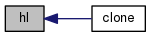Foam::scalar Cp ( scalar p, scalar T ) const
inlinevirtual

Liquid heat capacity [J/(kg K)].

Reimplemented from liquidProperties.

Definition at line 44 of file IDEAI.H.

References NSRDSfunc0::f().

Referenced by IDEA::clone().

Here is the call graph for this function: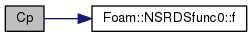Here is the caller graph for this function: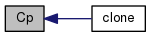Foam::scalar h ( scalar p, scalar T ) const
inlinevirtual

Liquid Enthalpy [J/(kg)].

Reimplemented from liquidProperties.

Definition at line 50 of file IDEAI.H.

References NSRDSfunc0::f().

Referenced by IDEA::clone().

Here is the call graph for this function: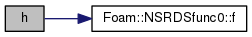Here is the caller graph for this function: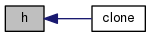Foam::scalar Cpg ( scalar p, scalar T ) const
inlinevirtual

Ideal gas heat capacity [J/(kg K)].

Reimplemented from liquidProperties.

Definition at line 56 of file IDEAI.H.

References NSRDSfunc7::f().

Referenced by IDEA::clone().

Here is the call graph for this function: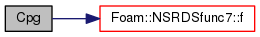Here is the caller graph for this function: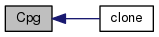Foam::scalar B ( scalar p, scalar T ) const
inline

Second Virial Coefficient [m^3/kg].

Definition at line 62 of file IDEAI.H.

References NSRDSfunc4::f().

Referenced by IDEA::clone().

Here is the call graph for this function: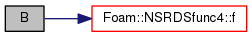Here is the caller graph for this function: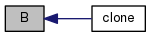Foam::scalar mu ( scalar p, scalar T ) const
inlinevirtual

Liquid viscosity [Pa s].

Reimplemented from liquidProperties.

Definition at line 68 of file IDEAI.H.

References NSRDSfunc1::f().

Referenced by IDEA::clone().

Here is the call graph for this function: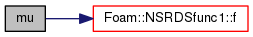Here is the caller graph for this function: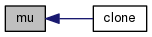Foam::scalar mug ( scalar p, scalar T ) const
inlinevirtual

Vapour viscosity [Pa s].

Reimplemented from liquidProperties.

Definition at line 74 of file IDEAI.H.

References NSRDSfunc2::f().

Referenced by IDEA::clone().

Here is the call graph for this function: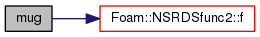Here is the caller graph for this function: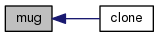Foam::scalar K ( scalar p, scalar T ) const
inlinevirtual

Liquid thermal conductivity [W/(m K)].

Reimplemented from liquidProperties.

Definition at line 80 of file IDEAI.H.

References NSRDSfunc0::f().

Referenced by IDEA::clone().

Here is the call graph for this function: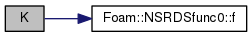Here is the caller graph for this function: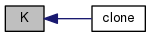Foam::scalar Kg ( scalar p, scalar T ) const
inlinevirtual

Vapour thermal conductivity [W/(m K)].

Reimplemented from liquidProperties.

Definition at line 86 of file IDEAI.H.

References NSRDSfunc2::f().

Referenced by IDEA::clone().

Here is the call graph for this function: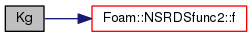Here is the caller graph for this function: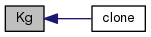Foam::scalar sigma ( scalar p, scalar T ) const
inlinevirtual

Surface tension [N/m].

Reimplemented from liquidProperties.

Definition at line 92 of file IDEAI.H.

References NSRDSfunc6::f().

Referenced by IDEA::clone().

Here is the call graph for this function: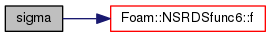Here is the caller graph for this function: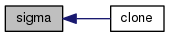Foam::scalar D ( scalar p, scalar T ) const
inlinevirtual

Vapour diffussivity [m2/s].

Reimplemented from liquidProperties.

Definition at line 98 of file IDEAI.H.

References APIdiffCoefFunc::f().

Referenced by IDEA::clone().

Here is the call graph for this function: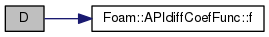Here is the caller graph for this function: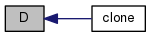Foam::scalar D ( scalar p, scalar T, scalar Wb ) const
inlinevirtual

Vapour diffussivity [m2/s] with specified binary pair.

Reimplemented from liquidProperties.

Definition at line 104 of file IDEAI.H.

References APIdiffCoefFunc::f().

Here is the call graph for this function:void writeData ( Ostream & os ) const
inlinevirtual

Write the function coefficients.

Reimplemented from liquidProperties.

Definition at line 195 of file IDEA.H.

Here is the call graph for this function: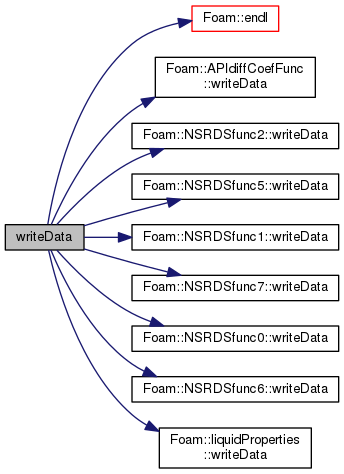## Friends And Related Function Documentation

 Ostream& operator<< ( Ostream & os, const IDEA & l )
friend

Ostream Operator.

Definition at line 213 of file IDEA.H.

The documentation for this class was generated from the following files:
• src/thermophysicalModels/properties/liquidProperties/IDEA/IDEA.H
• src/thermophysicalModels/properties/liquidProperties/IDEA/IDEA.C
• src/thermophysicalModels/properties/liquidProperties/IDEA/IDEAI.H